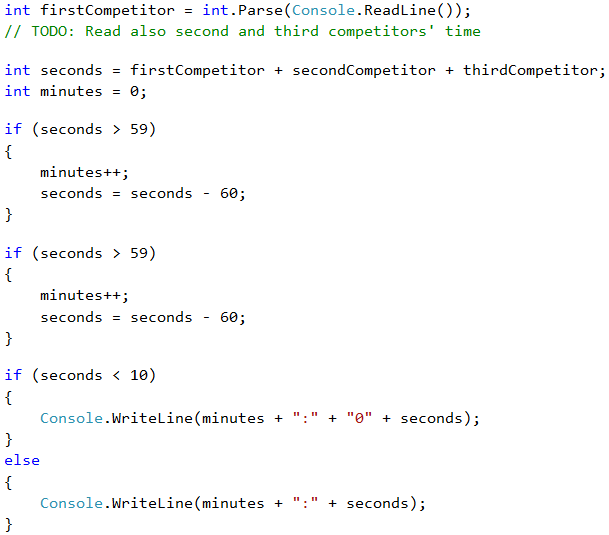# Problem: Summing Up Seconds

Three athletes finish in a particular number of seconds (between 1 and 50). Write a program that introduces the times of the contestants and calculates their total time in "minutes:seconds" format. Seconds need to be zeroed at the front (2 -> "02", 7 -> "07", 35 -> "35").

Input Output
35
45
44
2:04
22
7
34
1:03
50
50
49
2:29
14
12
10
0:36

## Video: Summing Up Seconds

Watch the video about the "Summing Up Seconds" problem and its solution: https://youtu.be/bnNxe79X-wo.

## Hints and Guidelines

First, we sum up the three numbers to get the total result in seconds. Since 1 minute = 60 seconds, we will have to calculate the number of minutes and seconds in the range 0 to 59:

• If the result is between 0 and 59, we print 0 minutes + calculated seconds.
• If the result is between 60 and 119, we print 1 minute + calculated seconds minus 60.
• If the result is between 120 and 179, we print 2 minutes + calculated seconds minus 120.
• If the seconds are less than 10, we print the number with zero in front.